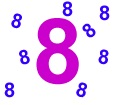When I went into a classroom earlier this week a child rushed up to tell me she was 8 that day!
Well, Happy Birthday to everyone who has a birthday today!
Whatever age you are, can you find a variety of questions that give your age as the answer? This could be 8 or any particular number you choose, for example 6+2, 22−14, etc.
But you need to get examples that use all the different mathematical ideas that you know about.
1) So you could show some multiplications and some divisions.
2) If you know about fractions then you can add or subtract numbers involving fractions. You could also ask questions like “What is half of 16?” , “What is four-fifths of 10?” and so on.
3) If you’ve come across decimals then do a few of those also, perhaps using all the four rules [addition, subtraction, multiplication and division].
And so on.
Use whatever mathematics you know to find as many different ways of getting the answer 8.
You may find some patterns that would go on for ever and ever. If you do, just put down a few, and then see if you can describe how the pattern works.
So if you’re 8 years old maybe you’ll write something like this:
16÷2, 8÷1, 4+4, 2+6, 9−1, 12−4
1+1+1+1+1+1+1+1, 2+2+2+2
15−3−2−1−1, 5+3+6−3−3
and so on.
Whatever your age, and whatever you get caught up with, have a look at the ways that you can make new ones that have a similar pattern.
Your “What would happen if …?” questions may be a little different from our usual ones.
The 8 year old might ask “I wonder what would happen if I tried to use multiplication and addition to make 8?”
But if you’re much much older you may write something like:-
4sin(π/2) + √(52 – 32)
Thanks to NRICH for this lesson activity. See AIMSSEC Teacher Notes I’M EIGHT

Tagged with: